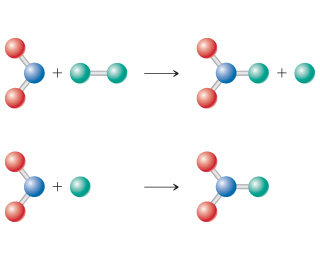# Problem: Consider the diagram below, which represents two steps in an overall reaction. The red spheres are oxygen, the blue ones nitrogen, and the green ones fluorine.a) Write the chemical equation for each step in the reaction.b) Write the equation for the overall reaction.

###### FREE Expert Solution

We're asked to write the equation for the overall reaction based on the given diagram.

We know that:

• red spheres are oxygen, O
• blue spheres are nitrogen, N
• green ones are fluorine, F

To do so, we need to do these steps:

Step 1.  Write the equation for each given reaction (two steps) based on the diagram

Step 2. Write the equation for the overall reaction by canceling out intermediates

Recall that intermediates are substances that are found on both sides of a chemical equation

81% (326 ratings)###### Problem Details

Consider the diagram below, which represents two steps in an overall reaction. The red spheres are oxygen, the blue ones nitrogen, and the green ones fluorine.a) Write the chemical equation for each step in the reaction.

b) Write the equation for the overall reaction.

Frequently Asked Questions

What scientific concept do you need to know in order to solve this problem?

Our tutors have indicated that to solve this problem you will need to apply the Reaction Mechanism concept. If you need more Reaction Mechanism practice, you can also practice Reaction Mechanism practice problems.

What professor is this problem relevant for?

Based on our data, we think this problem is relevant for Professor Daniel's class at Bakersfield College.